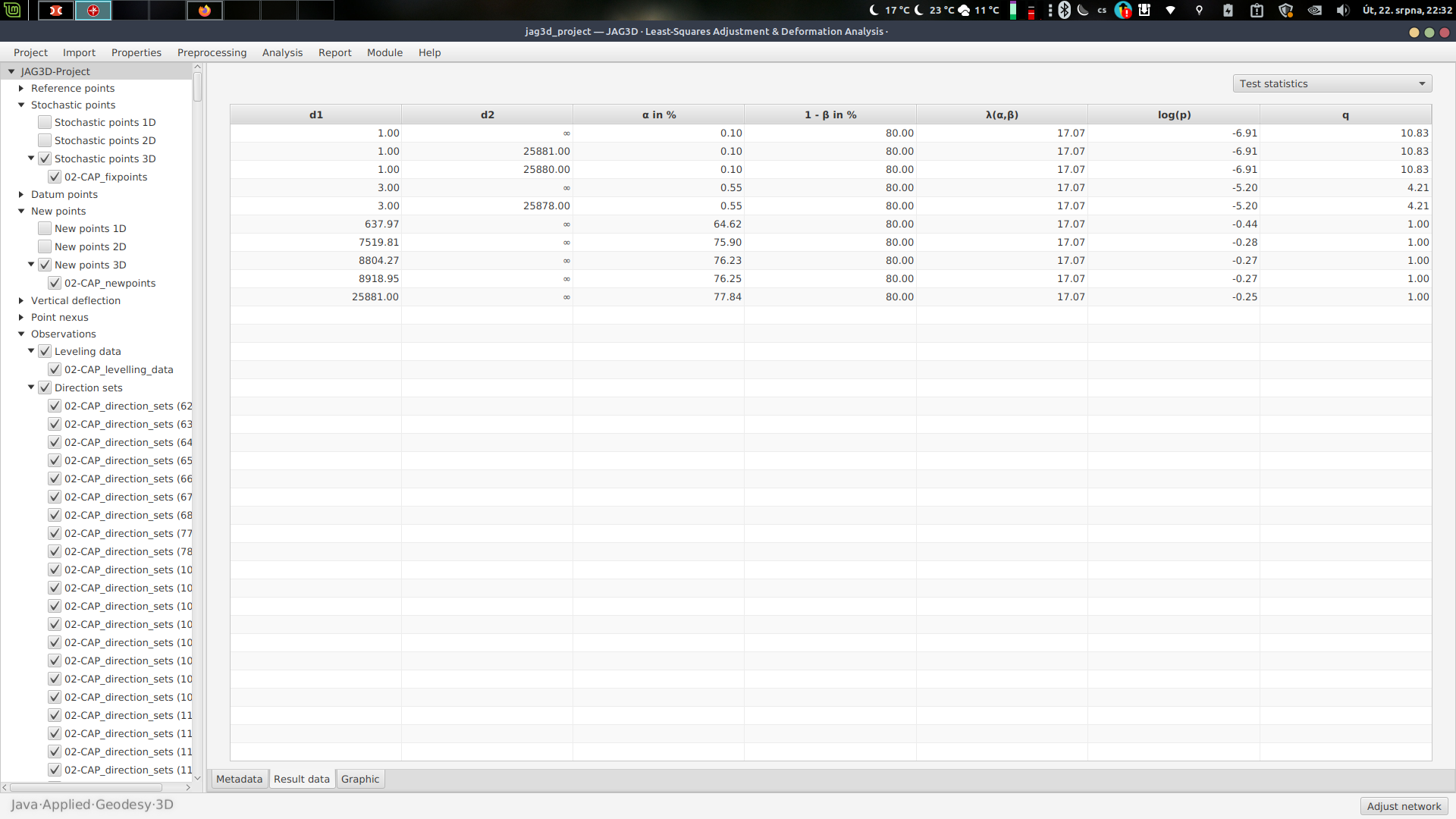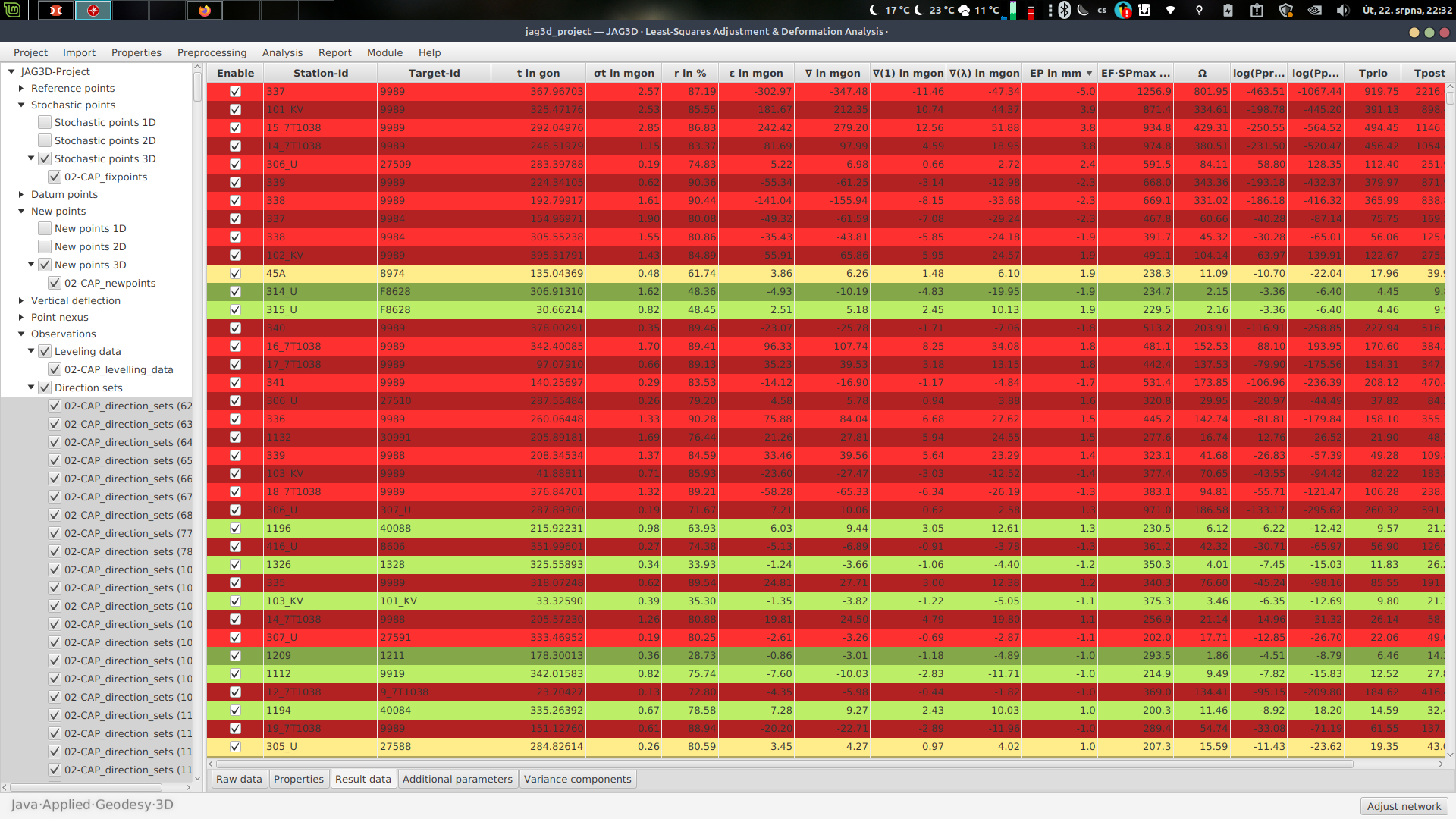# P-value meaning + rows highlighting

Hello Vlastimil,

As you wrote in the handbook, the P-value is logarithm of probability, that the individual observation belongs to the base population. If this logarithm is lower, than logarithm of the significance level alpha, then the zero hypothesis should be rejected (= the observation did not belong to the base population/observations set). Is this correct, please?

Yes, that sounds right to me. You can strip the logarithm from the interpretation. If $p < \alpha$, the null hypothesis is rejected.

Thanks for link, I have something to study. Based on the link - does JAG3D calculate the P-value for testing the Tprio/Tpost values?

As far if it is correct, then there is not clear, how the rows highlighting in accordance to P-value works. The previous statement splits the interval of possible results into two parts only - the part above significance level logarithm, where is the zero hypothesis valid, and the part under the significance level logarithm, where the zero hypothesis should be rejected. But in row highlighting settings is at the P-value two predefined values, which means the interval of possible values is splitted into three parts, instead of two.

Yes, that is right. The boundary values are taken from the Cadastre (in Germany). Here, 1 % and 5 % are used. For this reason, I have defined these values as default - but you are free to choose your own values.

Then the highlighting settings are mosty about significance level alpha, instead of the probability value in %. Isn't it there an misinterpretation of label? Shouldn't it be there in highlight settings written "significance level alpha in %" (respectively "Probability value alpha in %", as it is written in settings of test statistics), instead?

Then I want also to be clarified the behavior of highlighting. The P-value highlighting is not matching the exact value of logarithm of significance level, which is for 0,1 % (= 0,001) = -6,91, there are some rows highlighted regardless the condition log(p) > log(alpha).

This sounds like a bug and I have to reproduce this behavior by my own creating an example. The pie-charts in the HTML report seem to be correct. However, currently I'm at a measurement campaign and out of office. So, it will take a few days until I can check my source code.

As an illustration of this behavior am I attaching two screenshots I took. In the General tab are calculated logarithms of alpha for observations as -6,91. Row highlighting is setted for 0,1 and 1 %. Then the row with station 45A should be - if the highlighting have the similar logic, as T-values highlighting - red as well (the T-values highlighting marks row, where any of Tprio/Tpost overstep the critical value).If I understand correctly and highlight settings of P-value should be setted according to significance level alpha, then should be:

Boundaries in %: 0 <= 0,1 <= 1 <= 100
Boundaries in absolute: 0 <= 0,001 <= 0,01 <= 1
Boundaries in logarithm: -inf <= -6,91 <= -4,61 <= 0

At mine case the ln(Pprio) = -10,70 for station 45A is in the first interval and should be marked in red color, instead of yellow.

Similar behavior can be spotted at station 1326 at the bottom part of picture - that row should be marked as red as well, since both values are below ln(0,001).

I am currently using version, marked as:
UI v20230316
DB v20230131
AC v20230228

Thanks!
Vlastimil

I will come back to this thread as soon as possible.

/Micha